# A/B testing 5个问题

Microsoft在《Trustworthy Online Controlled Experiments: Five Puzzling Outcomes Explained 》中对A/B testing中存在的5个谜题做了解释。

# 3.1 搜索引擎的OEC

## 3.1.3 解释

$\frac{Users}{Month} \times \frac{Sessions}{User} \times {Distinct \ queries}{Session}$

…(1)

• 1.User per month。在一个controlled experiment中，独立用户数由设计决定。例如，在一个相等的A/B test中，用户数会以近似相同的数目分到两个variants中。（如果在variants中用户的比例很不同，这很可能是个bug）。出于该原因，该项不能成为该实验OEC的一部分。
• 2.每任务查询数（distinct queries per task）应该最小化，但它很难测量。每session不同查询数（distinct queries per session）是一个可用的代理指标(surrogate metric)。这是一个精细指标（subtle metric），然而，由于增加它可能意味着用户需要发起理多查询来完成该任务，但减少它可能表示放弃查询。该指标需要最小化，意味着该任务会被成功完成

## 3.1.4 学到的

query量的分解，搜索的长期目标，展示了冲突的组成(components)：一些会增加短期指标（sessions/user），另一些会减小短期指标（queries/session）表示任务成功完成。我们的假设是，更好的用户体验会增加users/month，最后一项不能在一个control实验中进行测量。

# 3.初始效应(Initial Effect)

## 3.3.2 Puzzling outcome## 3.3.3 解释• 1.相对于之前实验发布的最终结果（它们会运行更长的时间），在初始几天的effects通常看起来过度正向或者负向。
• 2.在前几天期间，累积结果看起来会延伸。例如，假设一个实验在兴趣指标上没有effect。头一天可能是负向的-0.6%，但随着更多数据被累计，该效应(effect)会回归到0均值、95%置信锥的true。feature owners不正确地假设该effect是会延伸的，那将很快会跨过0线。当然，这很少会发生。# 3.4 实验长度（Experiment Length）和统计强度(Statistical Power)

## 3.4.2 Puzzling Outcome# 3.5 延滞效应（carryover effects）

## 3.5.3 解释

“bucket system”的一个大缺陷是，它具有carryover effects的缺点，受前一实验影响的相同用户，被用于接下来的实验中。这是已知的，可以运行A/A tests确认carryover effects，但当他们失败时，我们会失去能力直到我们对bucket assignment进行re-randomize。令人吃惊的是，craayover effect的持续时间（duration）。我们分享以下的两个示例。## 3.5.4 缓解技术# A/B testing 5个问题

Microsoft在KDD2007时的paper《Practical Guide to Controlled Experiments on the Web:Listen to Your Customers not to the HiPPO》中，对A/B testing系统设计有一些practical guide，可以参考一下：

# 3.controlled experiments

## 3.1 术语

• OEC
• Factor
• Variant
• Experimentation Unit
• Null Hypothesis
• Confidence level
• Power
• A/A Test
• Standard Deviation (Std-Dev)
• Standard Error (Std-Err)

## 3.2 Hypothesis Testing和Sample Size

• 置信等级（confidence level）。通常设置为95%，该level表示：5%的机会是不正确的
• 统计功效（Power）。通常在80-95%间，尽管不是直接控制的。如果零假设是false的（比如：在OEC上有差异）, power表示决定该差异的概率，是统计显著的。（Type II error）
• (Standard Error)。Std-Err越小，test越强。有三种方法减小std-err：
• 被估计的OEC通常是大样本的一个平均。如3.1所示，一个均值(mean)的Std-Err会随着sample size的均方根按比例递减，因此，增大sample size（这通常意味着运行实验更久），可以减小Std-Err，从而增大power
• 使用天然具有更小变动性的OEC components（例如：Std-Dev, $\delta$），std-err会越小. 例如，转化率（conversion probability 0-100%）通常比购买单元数目（通常是小整数）具有更低的Std-Dev，换句话说，它比收益（revenue: real-valued）具有一个更低的std-dev。
• 通过过滤掉那些没有曝光给variants的用户，OEC的可变性会越低. 例如，如果你对checkout page做出一个变更，只分析访问该page的用户，由于每个人都增加了噪声(noise)，会增加可变性（variability）
• 4.the effect：对于variants来说在OECs上的差异。差异越大越容易检测，因此，great ideas不可能错过。相反的，如果Type I或Type II errors发生，当该effects很小时，他们更可能发生。

$n = (4r\delta / \Delta)^2$

• n是sample size
• r是variants数目
• $\delta$是OEC的std-dev
• $\Delta$是OECs之间的最小差异
• 4是因子，表示对于大的n会估计过高25%，但该近似会满足下面示例。

## 3.3 online settings的扩展

basic controlled experiments的一些扩展在online setting中是可能的。

## 3.4 限制

• 2.短期效应(short term) vs. 长期效应（long term effects）。controlled experiments会在实验期间（通常数周）衡量OEC的effect。而一些paper作者则批评说：这样只会注某一指标意味着short-term focus，我们对此不赞同。long-term目标应该是OEC的一部分。假设我们以搜索广告为例。如果你的OEC是收益（revenue），你可能在一个页面上粘帖数个广告，但我们知道：越多广告会伤害用户体验，因此一个好的OEC应包含对于没有点击的房地产广告的一个惩项（penalty term），或／和 对重复访问和放弃的直接measure。同样的，查看一个延迟的转化指标是明智的，其中从一个用户被曝光某样东西到该用户做出动作这段时间内有个迟延(lag)。这有时被称为潜在转化（latent conversions）。找好好的OEC是很难的，但有什么替代方法吗？这里的关键是，认识到该限制。
• 3.首效应(Primacy effects)和新效应（newness effects）。有一些负面效应需要认识。如果你在一个web网站上更换了导航，体验的用户可能更低效，直到他们完全适应了新导航，因而Control组会天然具有优势。相反的，如果一个新设计或新特性被引入，一些用户可能会研究它，到处进行点击，从而引入一个“newness” bias。该bias有时会与霍索恩效应（Hawthorne effect）相关。Primacy和Newness这两者意味着：一些实验需要运行多周。可以做个分析：为不同variants上的新用户计算OEC，因为他们不会受两个因素影响。
• 4.必须实现的特性。一个真实的controlled experiment需要曝光一些用户给一个Treatment，不同于当前站点（Control组）。该prototype可能是个prototype，它可以在很小比例上进行测试，或者不会覆盖所有的边缘情况（edge cases）（例如：实验可能故意排除20%的需要显著测试的浏览器类型）。尽管如些，该特性必须被实现，并曝光给用户足够的量。Jacob(34)正确指出：paper prototyping可以被用于在早期定性反馈和快速改进设计。我们赞同并推荐这样的技术来完善controlled experiments。
• 6.并行实验（Parallel Experiments）。我们的实验实际上很少发生很强的交叉（33），我们认为这一点是过度关注了。提升对这一点的关注对于实验者来说足够避免交叉（interact）的测试。成对的统计测试（statistical test）也可以完成，来自动标记这样的交叉（interactions）。
• 7.启动事件和媒体公告。如果对新特性做出一个大的公告，（比如：该特性通过媒体进行公告），所有用户需要看到它。

# 4.多因子实验(Multi-Variable Testing)

• 1.你可以在一个较短时间内测试许多factors，加速提升。例如，你想测试在网站上的5个变化，你需要每个A/B test运行4周时间来达到你需要的power，它会至少需要5个月来完成A/B tests。然而，你可以使用所有5个factors来运行单个MVT，它只需要1个月就可以达到5个A/B tests相同的power。
• 2.你可以估计factors间的交叉。两个factors的交叉，如果它们的组合效应与两个单独的effects叠加不同。如果这两个factors一起可以增强结果，那么该交叉（interaction）是协同的（synergistic）。如果相反的，他们相互抵触会抑制该效果，该交叉（interaction）是对抗的（antagonistic）。

• 1.一些factors的组合可能会给出一个较差的用户体验。例如，对于一个在线零售商来说，正在测试的两个factors可能是：增加一个商品的图片 or 提供额外的商品详情。当单独进行测试时，两者分别均会提升销售，但当两者在同时完成时，“buy box”会被推到折叠（fold）下面，这会减小销售。这就是一个大的对抗交叉（antagonistic interaction）。该交叉在计划阶段（planning phase）可以被捕获，以便这两个factors不会被同时测试。
• 2.分析和解释是更困难的。对于单个factor的test，你通常具有许多指标来进行Treatment-Control的比较。对于一个MVT，你需要为多个Treatment-Control比较设置相同的matrics（对于每个测试的factor至少要有一个），以及在factors间交叉的分析和解释。确定的是，该信息集越丰富，对于treatments的评估也越复杂。
• 3.它会花费更长时间开始测试。如果你具有希望测试的5个factors，并计划以一次测一个的方式测试它们，你可以启动任意待测的，接着测试其它的。使用一个MVT，你必须在test开始之前，具备所有5个准备好的testing。如果任何一个延误了，会延误test的启动。

# 5.实验架构

## 5.1 随机算法

• 1.用户必须等可能地看到一个实验的每个variant（假设：50-50 split）。对于任意指定variant必须是无偏的(no bias)。
• 2.对于单个user重复分配（repeat assignments）必须是一致的（consistent）；在对该网站的每次后续访问上，该user必须被分配到相同的variant上。
• 3.当多个实验同时并行运行时，实验之间必须没有关联（correlation）。在一个实验中，一个user被分配（assignment）到一个variant，对于被分配给其它任意实验的一个variant，在概率上没有影响
• 4.该算法必须支持单调递增（monotonic ramp-up），这意味着，如果没有对那些已经被配给Teatment的用户分配进行变更，用户看到一个Treatment的百分比可能会缓慢增加。

## 5.2 Assignment方法

Assignment方法是软件的一部分，它允许正实验的网站对于不同的用户执行不同的代码路径。有许多方法来实验一个assignment方法，优点和缺点各不同。

# 6.学到的课程

• (i) 分析
• (ii) trust & execution

# 2.相关工作

## 2.3 上下文个性化

• (a) Liu提出了 a social-aided context-aware recommender systems for books and movies. 会利用丰富的上下文信息来将user-item矩阵划分为多个矩阵
• (b) Jamali等人提出了: a context-dependent matrix factorization model to create user profiles for recommendation in social network 。

## 2.4 行为因子分解

“如果你将知觉（perception）看成是一种联系（contact）和洽谈(communion)的方式，那么，支配感知就是支配联系，限制和管理感知就是限制和管理联系。”—Erving Goffman, The Presentation of Self in Everyday Lif.

## 3.2 衡量行为间的不同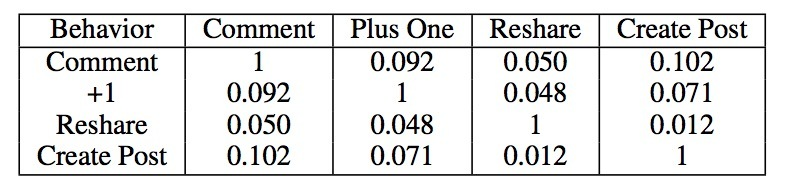# 4.问题定义

## 4.1 输入行为信号

$I = \lbrace t_i = (u_i, b_i, E_i), i=1, ..., N \rbrace$

• 每个$t_i$表示一个用户在社交媒体内容的某个特定片段上的动作。例如，一个$t_i$可以是：创建一个post，或者对某个post进行comment。
• $E_i$是该post的特征集合。

## 4.2 User profiles

$B = \lbrace P_u = \lbrace V_{u_B} \rbrace \rbrace$

• $P_u$是用户u的user profile，
• $V_{u_B}$是用户u在对应于她的行为类型B上的偏好向量。

$P_u$可以被认为是一个user tensor。

B即可以是单个行为类型（例如：创建一个post），或是一个不同行为类型的组合（例如：创建一个post和reshare一个post组合）。准确表述为：

$V_{u_B} = ( p_{u_B}^{e_1}, p_{u_B}^{e_2}, ..., p_{u_B}^{e_k} ), e_j \in E$

# 5.我们的方法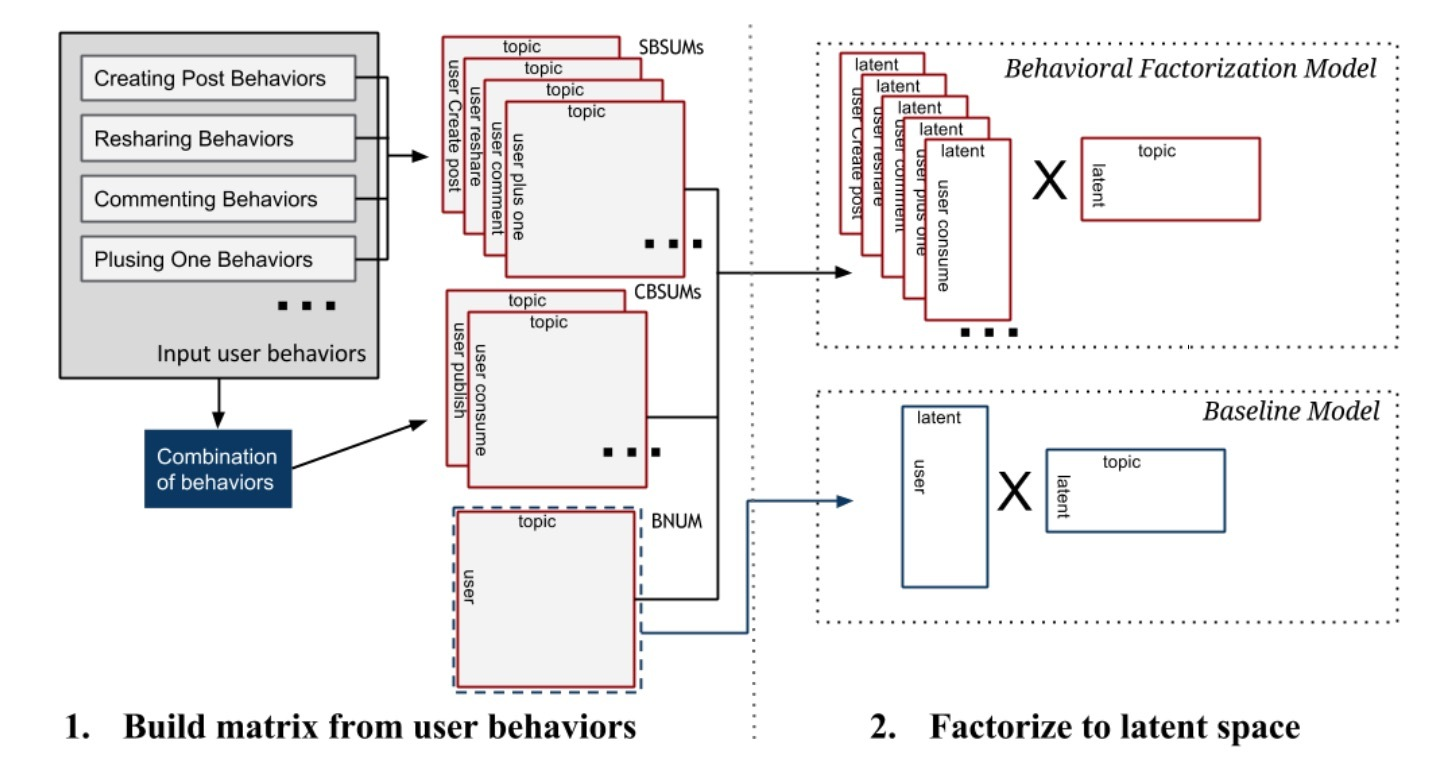• step 1: 给定第4节中定义的input user action tuples $I$，我们首先构建不同行为类型的矩阵。这对应于图1中的左部分。
• step 2: 我们对step 1中生成的矩阵进行因子分解来学到latent embedding space。这对应于图1中的右部分。
• step 3: 最后，我们使用学到的latent space来对兴趣主题做预测来构建user profiles。这会为每个用户u创建profiles $P_u = \lbrace V_{u_B} \rbrace$。这对应于图2.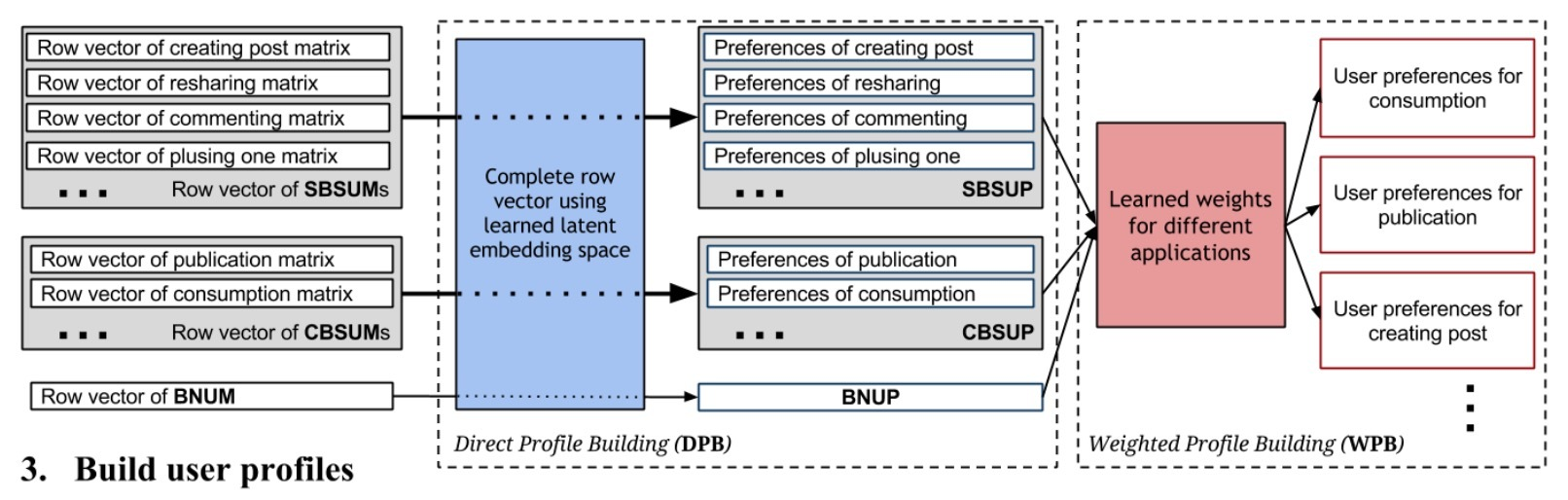## 5.1 step 1: 为不同行为类型构建矩阵

• (a) 传统的$N \times K$矩阵，被称为Behavior Non-specific User-topic Matrix(BNUM)
• (b) Single Behavior-Specific User-topic Matrix(SBSUM)
• (c) Combined Behavior-Specific User-topic Matrix(CBSUM)

### 5.1.1 BNUM (Behavior Non-specific User-topic Matrix)

$I_u = \lbrace t_j = (u_j, b_j, E_j) \rbrace, t_j \in I \wedge u_j = u$

$r_{ui} = r(I_u, i)$

$r_{ui} = \frac{(\sum\limits_{I_u} \sum\limits_{e \in E_j} \sigma_i(e)) + 1}{(\sum\limits_{I_u} \| E_j \|) + (\|\cup_{I_u} E_j\|)}$

…(1)

### 5.1.2 SBSUM (Single Behavior-Specific User-topic Matrix SBSUM)

SBSUM和CBSUM将行为类型单独划分来生成独立的user-topic矩阵。给定一个行为类型的特征集合$B \subset B$，我们想构建矩阵$R_B = \lbrace r_{ui}^B \rbrace$，其中每个条目表示从B中行为类型得到的隐式兴趣。

$r_{ui}^B = \frac{(\sum\limits_{I_u \wedge b_j \in B} \sum\limits_{e \in E_j} \sigma_i(e)) + 1}{ \sum\limits_{I_u \wedge b_j \in B} \| E_j \| ) + (\|\cup_{I_u \wedge b_j \in B} E_j \|)}$

…(2)

$\begin{cases} R_{b_1} = \lbrace r_{ui}^B \rbrace, B=\lbrace b_1,b_1 \in \mathscr{B} \rbrace \\ R_{b_2} = \lbrace r_{ui}^B \rbrace, B=\lbrace b_2,b_2 \in \mathscr{B} \rbrace \\ ... \\ R_{b_M} = \lbrace r_{ui}^B \rbrace, B=\lbrace b_M,b_M \in \mathscr{B} \rbrace \end{cases}$

…(3)

### 5.1.3 CBSUM（Combined Behavior Specific User-topic Matrix）

$\begin{cases} R_{B_1} = \lbrace r_{ui}^B \rbrace, B=\lbrace B_1,B_1 \in \mathscr{B} \rbrace \\ R_{B_2} = \lbrace r_{ui}^B \rbrace, B=\lbrace B_2,B_2 \in \mathscr{B} \rbrace \\ ... \\ R_{B_M} = \lbrace r_{ui}^B \rbrace, B=\lbrace b_M,b_M \in \mathscr{B} \rbrace \end{cases}$

…(4)

## 5.2 step 2: 学习latent embedding space

### 5.2.1 baseline: MF

$p_{ui} = \begin{cases} 1 , r_{ui}>0 \\ 0 , r_{ui}=0 \end{cases}$

$\min\limits_{x_*,y_*} \sum\limits_{ui} c_{ui} (p_{ui} - x_u^T y_i) ^ 2 + \lambda( \sum\limits_{u} \| x_u \|^2 + \sum\limits_i \| y_i \|^2)$

…(5)

### 5.2.2 行为因子分解模型（BF）

$min_{x_*,y_*} \sum\limits_{B \in \Gamma} \sum\limits_{u,i} c_{ui}^B (p_{ui}^B - x_u^{B^T} y_i)^2 + \lambda (\sum\limits_{B \in \Gamma} \sum\limits_{u} \| x_u^B \|^2 + \sum\limits_{i} \|y_i\|^2)$

….(6)

## 5.3 step 3: 构建user profiles

• i) 从profile矩阵的input row vectors构建direct profile
• ii) 通过一个回归模型学到一个权重集合，合并不同的direct profile进行构建weighted profile

• BNUP:
• SBSUP:
• CBSUP:

### 5.3.1 DPB(Direct Profile Building)

$P_u = \lbrace V_{u_B} = x_u^{B^T} Y \brace, B \in \Gamma$

…(7)

$V_{u_B} = ( p_{u_B}^{e_1}, p_{u_B}^{e_2}, ..., p_{u_B}^{e_K}), p_{u_B}^{e_i} = x_u^{B^T} y_1$

…(8)

$V_u = (p_u^{e_1}, p_u^{e_2}, ..., p_u^{e_K}), p_u^{e_i} = x_u^T y_i$

…(9)

### 5.3.2 WPB (Weighted Profile Building)

DPB会为一个用户生成一个behavior profile（如果该用户在过往有行为）。通过将用户的行为类型相分隔，我们可以使用DPB来为用户u的行为B生成profile，但这需要用户u在行为B上具有非零的观察值。对于那些在B上没有行为的用户，$V_{u_B}$会为空，这意味着一个没有该行为类型动作的user，不会有一个user profile。这在某种程度上对应于在推荐系统中的冷启动问题。

$V_{u_B} = \sum\limits_{B_t \in \Gamma} W_{B_t} x_u^{B_t^T} Y$

…(10)

$\Gamma$中不同行为类型的权重是模型级别的参数，例如：我们会为整个数据集的每个$B_t \in \Gamma$学到一个权重。因此，这些权重可以使用一个监督学习方法来从在我们的数据集中具有多种行为类型的所有用户上学到。因此，对于那些在过程历史记录中没有$B_t$的用户，我们仍可以为他们构建profiles。

# 6.评估

• H1: 从我们的行为因子分解方法中学到的latent embedding模型在构建user profiles比baseline MF模型要更好
• H2: 通过从多种行为类型中组合偏好向量，我们可以提升在特定行为类型上的user profiles的覆盖度(coverage)

## 6.1 实验设置

### 6.1.1 Dataset

• SBSUM
• Publication CBSUM
• Consumption CBSUM
• BNUM

### 6.1.2 评估指标

• Recall@N: $L_{method}$的top N的主题有多少出现在$L_{observed}$
• NDCG@N:
• AP@N:

# 2.二元离散化

$Ent(S) = - \sum_{i=1}^{k} P(C_i,S) log(P(C_i,S))$

$E(A,T;S) = \frac{|S_1|}{|S|} Ent(S_1) + \frac{|S_2|}{|S|} Ent(S_2)$

…(2)

A的二分离散化通过选择分割点$T_A$来决定，其中$E(A, T_A; S)$是所有候选分割点中的最小值。

## 2.1 分割点选择的讨论# 3.泛化该算法## 3.1 分割还是不分割？

$B = c_{11} Prob(d_A \wedge HT) + c_{22} Prob (d_R \wedge NT) + c_{12} Prob(d_A \wedge NT) + c_{21} Prob (d_R \wedge HT)$

## 3.2 MDLP(最小描述长度原则)

MDLP原则不必要要求与之前讨论的决策原则不同。它可以轻易地展示MDLP和Bayesian risk minimization strategy在理论上是相互相关的。由于篇幅原因，我们忽略了派生版本，它包含着可以包含对数据集S的hypothesis H所需要指定的bits数：$-log_2 (Prob(H|S))$，使用Bayes’ rule。最终获得的表达式等价于MDLP。这可以看成是采用MDLP的动机。

## 3.3 应用MDLP：编码问题

$log_2(N-1) + | S_1 | \cdot l_1 + | S_2 | \cdot l_2$ (bits)

$G_k = [ \sum_{k_1=1}^{k-1} (C_{k_1}^{k}) 2^{k_1}] + 2^k - 1 = 3^k - 2$

##

# 介绍

《BPR: Bayesian Personalized Ranking from Implicit Feedback》讨论了个性化排序学习模型的一个通用方法：Bayesian Personalized Ranking。主要贡献有：

• 1.描述了通用的优化方法：BPR-OPT，它来自于对最优个性化排序的最大后验估计。我们展示了BPR-OPT在AUC上的分析。
• 2.对于最大化BPR-OPT，我们提出了通用学习算法LearnBPR，它基于SGD，在训练过程使用bootstrap sampling。我们展示了该算法会优于最优化BPR-OPT时的SGD。
• 3.我们展示了如何应用learnBPR到两个state-of-art推荐模型中
• 4.我们的实验经验上展示了个性化排序任务，使用BPR的模型效果要好于其它学习算法

。。。

# 3.个性化排序（Personalized Ranking）

## 3.1 公式化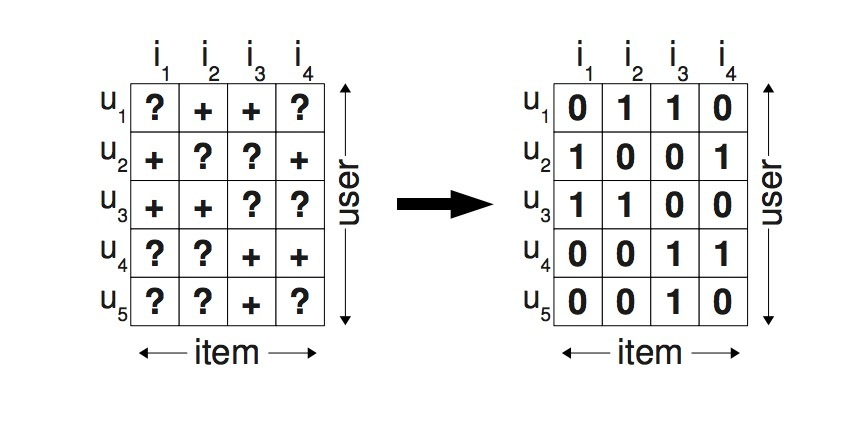\begin{aligned} & \forall i,j \in I: i \neq j \Rightarrow i >_u j \vee j >_u i \ \ (totality) \\ & \forall i,j \in I: i >_u j \wedge j >_u i \Rightarrow i = j \ \ (antisymmetry) \\ & \forall i,j \in I: i >_u j \wedge j >_u k \Rightarrow i >_u k \ \ (transitivity) \end{aligned}
• totallity: 总体性
• antisymmetry: 反对称性
• transitivity: 传递性

$I_u^+ := {i \in I: (u,i) \in S} \\ U_i^+ := {u \in U: (u,i) \in S}$

## 3.2 问题分析

• 正例：$(u, i) \in S$ pairs
• 负例：所有在$(U \times I) \backslash S$中的其它组合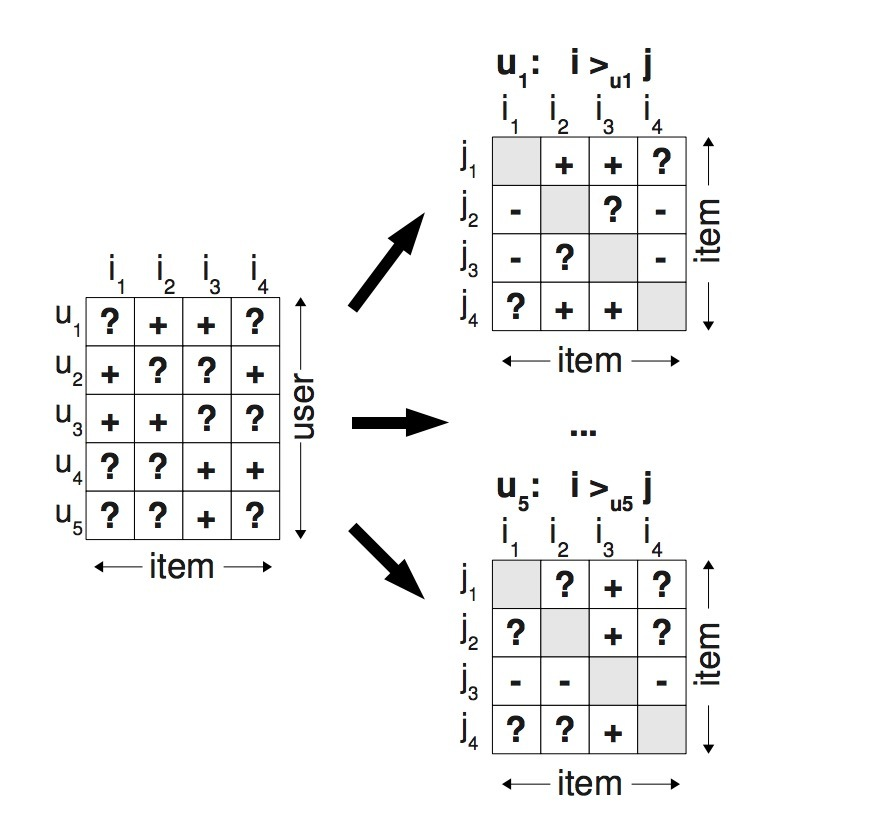$D_S := \lbrace (u, i, j) | i \in I_u^+ \wedge j \in I \backslash I_u^+ \rbrace$

$(u,i,j) \in D_S$的语义是，user $u$被假设成：喜欢i，胜过j。由于$>_u$是非对称的，负例会被隐式对待。

• 1.我们的训练数据同时包含了正负例pairs以及缺失值。介于两个未观察到的items间的缺失值是将来必须排序的item pairs。这意味着，从pairwise的角度看，训练数据$D_S$和测试数据是不相交的。
• 2.为排序的实际目标函数创建训练数据，例如：观察到$>_u$的子集$D_S$被用成训练数据。

# 4.BPR

## 4.1 BPR优化原则

$P(\Theta | >_u) \propto p(>_u | \Theta) p(\Theta)$

$\prod\limits_{u \in U} p(>_u | \Theta) = \prod\limits_{(u,i,j) \in U \times I \times I} p(i >_u j | \Theta)^{\delta((u,i,j) \in D_S)} \cdot(1-p(i >_u j | \Theta))^{\delta((u,j,i) \notin D_S}$

$\delta(b) := \begin{cases} 1 & \text{if b is true,} \\ 0 & \text{else} \end{cases}$

$\prod\limits_{u \in U} p(>_u | \Theta) = \prod\limits_{(u,i,j) \in D_S} p(i >_u j | \Theta)$

$p(i >_u j | \Theta) = \sigma( \hat{x}_{uij} (\Theta))$

• $\sigma$是logistic sigmoid：$\sigma(x) := \frac{1}{1+e^{-x}}$
• $\hat{x}_{uij}(\Theta)$是一个特定的关于模型参数向量$\Theta$的real-valued函数，它会捕获user u、item i、item j间的特殊关系。

$p(\Theta) \sim N(0, \sum_{\Theta})$

\begin{aligned} BPR-OPT &:= ln \ p(\Theta | >_u) \\ & = ln \ p(>_u | \Theta) p(\Theta) \\ & = ln \ \prod\limits_{(u,i,j) \in D_S} \sigma(\hat{x}_{uij}) p(\Theta) \\ & = \sum\limits_{(u,i,j) \in D_S} ln \ \sigma(\hat{x}_{uij}) + ln \ p(\Theta) \\ & = \sum\limits_{(u,i,j) \in D_S} ln \ \sigma(\hat{x}_{uij}) - \lambda_{\Theta} \|\Theta \|^2 \end{aligned}

### 4.1.1 AUC最优化分析

$AUC(u) := \frac{1}{ | I_u^+ | |I \backslash I_u^+ |} \sum\limits_{i \in I_u^+} \sum\limits_{j \in | I \backslash I_u^+|} \sigma(\hat{x}_{uij} > 0)$

$AUC := \frac{1}{|U|} \sum\limits_{u \in U} AUC(u)$

…(1)

$z_u = \frac{1} { | U | | I_u^+ | | I \backslash I_u^+|}$

$\sigma(x > 0) = H(x) := begin{cases} 1, & \text{ x > 0 } \\ 0, & \text{ else } end{cases}$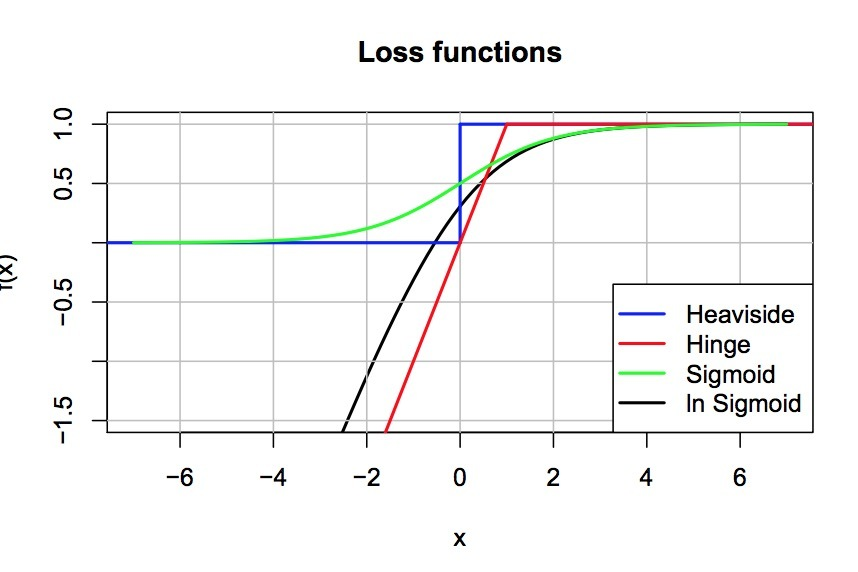## 4.2 BPR learning算法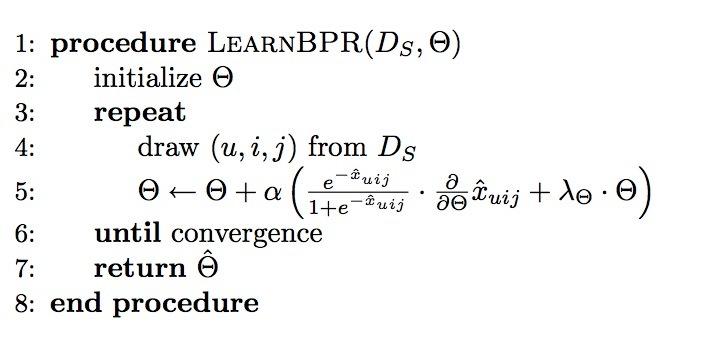\begin{aligned} \frac{\partial {BPR-OPT}}{\partial \Theta} & = \sum_{(u,i,j) \in D_S} \frac{\partial}{\partial \Theta} ln \sigma(\hat{x}_{uij}) - \lambda_{\Theta} \frac{\partial}{\partial \Theta} \| \Theta \|^2 \\ & \propto \sum\limits_{(u,i,j) \in D_S} \frac{-e^{-\hat{x}_{uij}}}{1+e^{-\hat{x}_{uij}}} \cdot \frac{\partial}{\partial \Theta} \hat{x}_{uij} - \lambda_{\Theta} \Theta \end{aligned}

$\theta \leftarrow \Theta - \alpha \frac{\partial BPR-OPT} {\partial \Theta}$

$\Theta \leftarrow \Theta + \alpha ( \frac{e^{-\hat{x}_{uij}}}{1+e^{\hat{x}_{uij}}} \cdot \frac{\partial}{\partial \Theta} \hat{x}_{uij} + \lambda_{\Theta} \Theta)$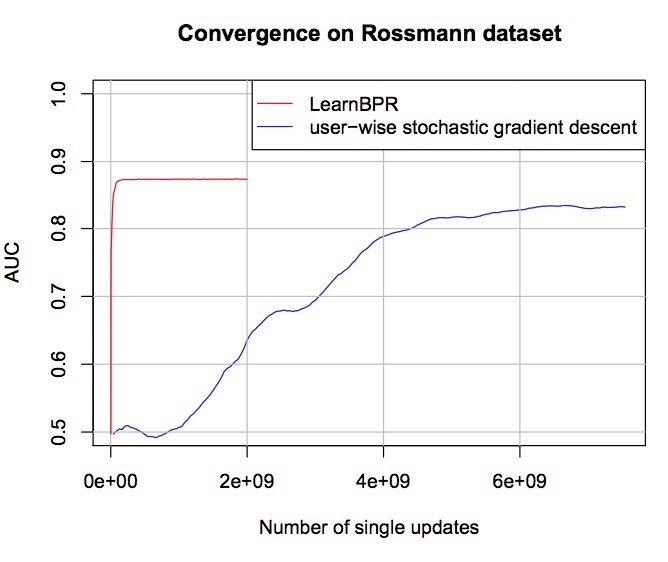## 4.3 使用BPR来学习模型

$\hat{x}_{uij} := \hat{x}_{ui} - \hat{x}_{uj}$

### 4.3.1 MF

$\hat{X} := W H^t$

$\hat{x}_{ui} = \langle w_u, h_i \rangle = \sum\limits_{f=1}^{k} w_{uf} \cdot h_{if}$

$\frac{\partial}{\partial \theta} \hat{x}_{uij} = \begin{cases} (h_{if} - h_{jf}) & \text{if \theta = w_{uf} } \\ w_{uf} & \text{if \theta = h_{if}} \\ -w_{uf} & \text{if \theta = h_{jf}} \\ 0 & \text{else} \end{cases}$

• $\lambda_W$对应用户特征W;

item features H有两个正则常数：

• $\lambda_{H^+}$被用于只在$h_{if}$上用于positive更新；
• $\lambda_{H^-}$用于在$h_{jf}$上的negative更新

$\hat{x}_{ui} = \sum\limits_{l \in I_u^+ \wedge l \neq i} c_{il}$

$c_{i,j}^{cosine} := \frac{|U_i^+ \cap U_j^+|} {\sqrt{ |U_i^+ | \cdot |U_j^+|}}$

$\frac{\partial}{\partial \Theta} \hat{x}_{uij} = \begin{cases} +1 & \text{if \theta \in \lbrace c_{il}, c_{li} \rbrace \wedge l \in I_u^+ \wedge l \neq i,} \\ -1 & \text{if \theta \in \lbrace c_{jl}, c_{lj} \rbrace \wedge l \in I_u^+ \wedge l \neq j,} \\ 0 & \text{else} \end{cases}$

# 5.与其它方法的关系

• WR-MF: Weighted Regularized Matrix Factorization
• MMMF: Maximum Margin Matrix Factorization

# 6.评估

## 6.1 datasets

• Rossmann dataset：来自在线电商。它包含了1w用户在4k items上的购买历史。总共有426612个购买记录。该任务是预测用户希望在下次购买的一个个性化items列表.
• Netflix DVD rental dataset: 该数据集包含了用户行为的打分，其中一个用户对电影提供了1-5星的显式评分。如果我们希望以隐式反馈的方式求解，我们可以移除rating。该任务是预测用户是否可能对一个电影进行评分。我们会为用户提供一个最可能打分的个性化排序列表。对于Netflix我们创建了一个subsample: 1w用户，5000 items，包含了565738个rating动作。我们会做子抽样：每个用户至少包含10个items，每个item至少有10个用户。

## 6.2 评估方法

$AUC = \frac{1}{|U|} \sum\limits_{u} \frac{1}{|E(u)|} \sum\limits_{(i,j) \in E(u)} \sigma(\hat{x}_{ui} > \hat{x}_{uj}$

…(2)

$E(u) := \lbrace (i,j) | (u,i) \in S_{test} \wedge (u,j) \notin (S_{test} \cup S_{train})rbrace$

…(2)

AUC越高表示效果越好。随机猜测法的AUC是0.5,最好为1。

## 6.3 结果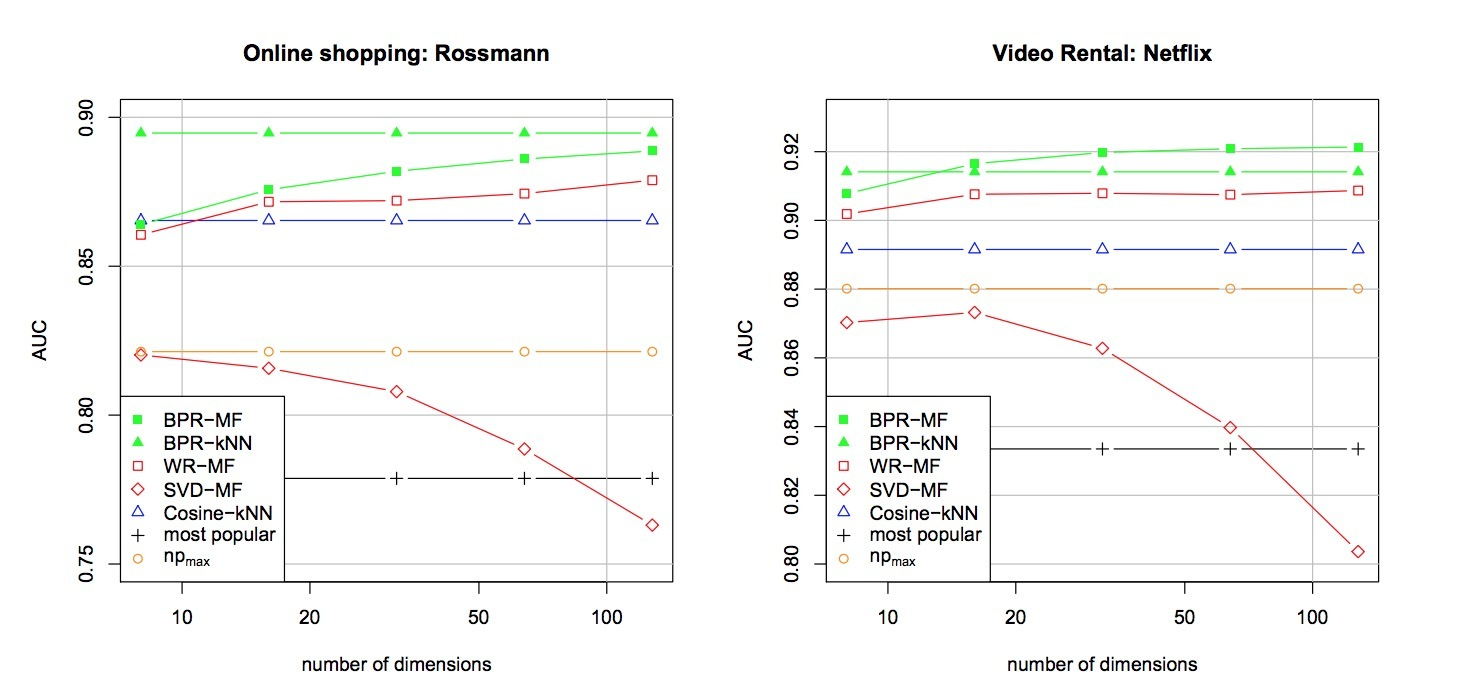# 参考

https://arxiv.org/pdf/1205.2618.pdf